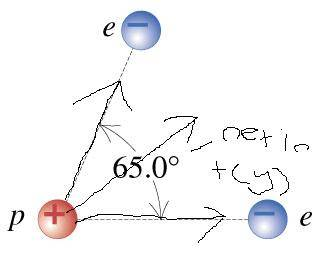# Picture to make sure my concepts are correct.

Mdhiggenz

## Homework Statementcan you judge the diagram I want to make sure i have the forces being exerted in the correct order.

Staff Emeritus
Homework Helper
Gold Member

## Homework Statementcan you judge the diagram I want to make sure i have the forces being exerted in the correct order.

## The Attempt at a Solution

That's the correct direction. If you add the vectors correctly, you will also get the correct magnitude !

Mdhiggenz
One more question sam. I have want to make sure I clearly understand, Let's call the top top electron E1, and bottom E2.

I only have to get the Cosine of the bottom election because it is on the horizontal and not on an angle, but for E1 I have to get both the sin and cos.

And to get the magnitude I can simply add squareroot fsin(theta)+2fcos(theta) ?

Staff Emeritus
Homework Helper
Gold Member
One more question sam. I have want to make sure I clearly understand, Let's call the top top electron E1, and bottom E2.

I only have to get the Cosine of the bottom election because it is on the horizontal and not on an angle, but for E1 I have to get both the sin and cos.

And to get the magnitude I can simply add squareroot fsin(theta)+2fcos(theta) ?
No. That won't give the correct answer.

There seems to be the assumption that the electrons are equidistant from the proton, so that the magnitude of the force that each electron exerts on the proton is f. Right?

What are you calling θ, (theta)?

If θ = 65°/2, then (f)cos(θ) the component of the force along the resultant that's exerted by either of the electrons.

Mdhiggenz
Pretty much my theta is 65 degrees and what I am doing is this

√(F)^2+(Fsin(65))^2+(Fcos(65))^2

and I don't understand how it isn't giving me the answer

Staff Emeritus
Homework Helper
Gold Member
Pretty much my theta is 65 degrees and what I am doing is this

√(F)^2+(Fsin(65))^2+(Fcos(65))^2

and I don't understand how it isn't giving me the answer
Earlier you said "squareroot fsin(theta)+2fcos(theta)".

But what you have here is also not correct.

The horizontal component is F + F cos(65°) which is equal to F(1 + cos(65°)) .

The vertical component is F sin(65°) .

Square each component, sum those & take the square root of the result. That's close to what you have, but (a + b)2 ≠ a2 + b2.

Staff Emeritus
Homework Helper
Gold Member
Pretty much my theta is 65 degrees and what I am doing is this

√(F)^2+(Fsin(65))^2+(Fcos(65))^2

and I don't understand how it isn't giving me the answer
Now that a complete answer to a similar, but more detailed problem has been given in another of your threads, I'll give the solution here, arrived at in two ways.

If F is the magnitude of the force that one of the electrons exerts on the proton, then:

E2 exerts a force of F in the horizontal direction.

E1 exerts a force which has a component of F cos(65°) in the horizontal direction and a component of F sin(65°) in the vertical direction.

The magnitude of that force is:

$\displaystyle \sqrt{\left(F+F\cos(65^\circ)\,\right)^2+\left(F \sin(65^\circ)\,\right)^2}$
$\displaystyle =F\sqrt{\left(1+\cos(65^\circ)\,\right)^2+\sin^2(65^\circ)}$

$\displaystyle =F\sqrt{1+2\cos(65^\circ)+\cos^2(65^\circ)+\sin^2(65^\circ)}$

$\displaystyle =F\sqrt{2+2\cos(65^\circ)}$

$\displaystyle =2F\sqrt{\frac{1+\cos(65^\circ)}{2}}$

$\displaystyle =2F\cos(32.5^\circ)$​

A simpler way:

Of course if you look at the component of the force E2 exerts parallel to the bisector of the 65° angle, that component is F cos(32.5°). Similarly the component of the force E1 exerts in that same direction is also F cos(32.5°). Making the resulting component of the force parallel to the bisector 2F cos(32.5°).

The forces perpendicular to the bisector cancel.

Mdhiggenz
Really appreciate the clear and thorough explanation. It makes perfect sense now!